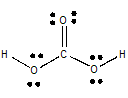# Is H2CO3 a polar molecule?

Aug 22, 2015

Yes, ${\text{H"_2"CO}}_{3}$ is a polar molecule.

#### Explanation:

The Lewis structure of ${\text{H"_2"CO}}_{3}$ is(from employees.csbsju.edu)

With respect to the carbon atom, this is an ${\text{AX}}_{3}$ system,

The geometry about the $\text{C}$ atom is trigonal planar, but the $\text{C=O}$ dipole is not balanced by the $\text{C-O}$ dipoles.

So the molecule is polar.